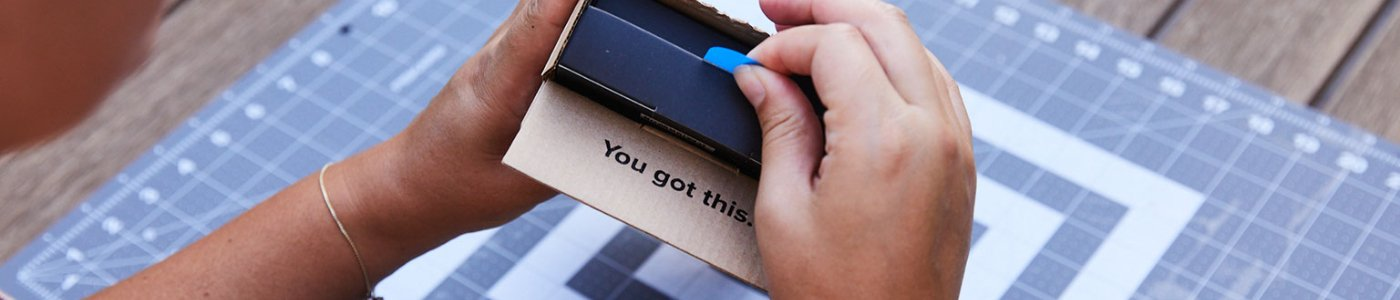跳转到主内容

## Chumby One

The Chumby One was released in late 2009.

1 个问题 查看全部

# I nedd some help

i need some help with my sons homework, lol what are these in order from least to greatest: 100%, 1/100, 0.001, 1.1, 10 , and 10000/100### 跳过升级

#### 通过使用多合一工具包维修可以节省成本。## 1个回答

Hi ,

.001 =1/1000

.01 = 1/100

1.1

10

100 =10000/100

100% I believe does not belong in the sequence because % (percent or per 100) is used to denote a part of a total amount expressed as a fraction of 100, e.g 3/4 expressed as a % = 75% (3 x 100 / 4=75).

As the denominator value of the 100% in your question is unknown we don't know whether 100% is greater or less than any of the other amounts stated, e.g. 100% of 1 =1, 100% of 50 = 50 and 100% of .01 =.01 so 100% of unknown value = unknown value

## 添加你的答案crazy derpy gamer 将永远感激不已